# Excel TOROW function to convert range / array to single row

A quick way to turn a range of cells into a single row with the help of the TOROW function.

Microsoft Excel 365 has introduced several new functions to perform various manipulations with arrays. With TOROW, you can perform range-to-row transformations in no time. Here is a list of tasks that this new function can accomplish:

## Excel TOROW function

The TOROW function in Excel is used to convert an array or range of cells into one row.

The function takes a total of three arguments, of which only the first one is required.

TOROW(array, [ignore], [scan_by_column])

Where:

Array (required) - an array or range to transform into a single row.

Ignore (optional) - determines whether to ignore blanks or/and errors. Can take one of these values:

• 0 or omitted (default) - keep all values
• 1 - ignore blanks
• 2 - ignore errors
• 3 - ignore blanks and errors

Scan_by_column (optional) - defines how to scan the array:

• FALSE or omitted (default) - scan the array horizontally by row.
• TRUE - scan the array vertically by column.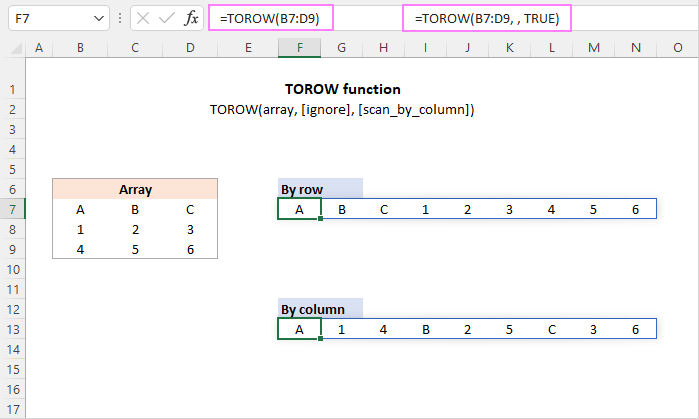Tips:

• To transform an array into a single column, utilize the TOCOL function.
• To preform the reverse row-to-array transformation, use either the WRAPCOLS function to wrap into columns or the WRAPROWS function to wrap array into rows.
• To turn rows into columns, use the TRANSPOSE function.

### TOROW availability

TOROW is a new function, which is only supported in Excel for Microsoft 365 (for Windows and Mac) and Excel for the web.

## Basic TOROW formula in Excel

To do a simple range-to-row transformation, use the TOROW formula in its basic form. For this, you need to define only the first argument (array).

For instance, to turn a two-dimensional array consisting of 3 columns and 3 rows into a single row, the formula is:

`=TOROW(A3:C6)`

You enter the formula into just one cell (A10 in our case), and it automatically spills into as many cell as needed to hold all the results. In Excel terms, the output range surrounded by a thin blue border is called a spill range.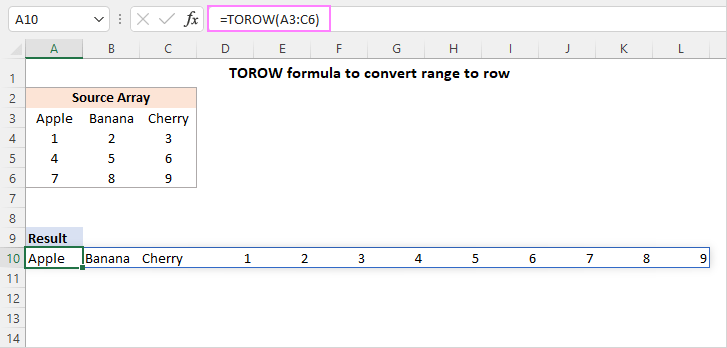How this formula works:

First, a supplied range of cells is transformed into a two-dimensional array. Please notice the comma-delimited columns and semicolon-separated rows:

`{"Apple","Banana","Cherry";1,2,3;4,5,6;7,8,9}`

Then, the TOROW function reads the array from left to right and converts it into a one-dimensional horizontal array:

`{"Apple","Banana","Cherry",1,2,3,4,5,6,7,8,9}`

The result goes to cell A10, from which it spills into the neighboring cell on the right.

## Transform range to row ignoring blanks and errors

By default, the TOROW function keeps all the values from the source array, including empty cells and errors. In the output, zero values appear in the place of blank cells, which may be quite confusing.

To exclude blanks, set the ignore argument to 1:

`=TOROW(A3:C5, 1)`

To ignore errors, set the ignore argument to 2:

`=TOROW(A3:C5, 2)`

To skip both, blanks and errors, use 3 for the ignore argument:

`=TOROW(A3:C5, 3)`

The image below shows all three scenarios in action: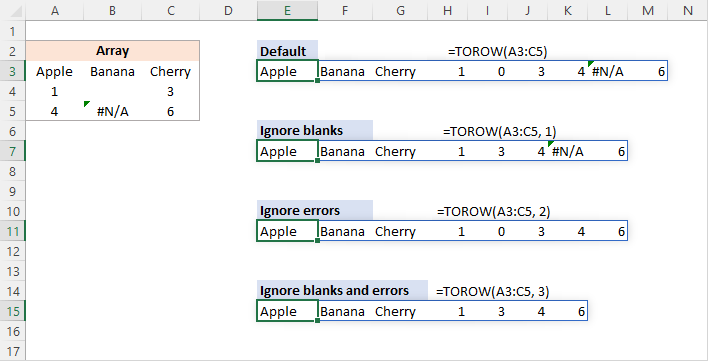## Read array horizontally or vertically

With the default behavior, the TOROW function processes the array horizontally from left to right. To scan the values by column from top to bottom, you set the 3rd argument (scan_by_column) to TRUE or 1.

For example, to read the source range by row, the formula in E3 is:

`=TOROW(A3:C5)`

To scan the range by column, the formula in E8 is:

`=TOROW(A3:C5, ,TRUE)`

In both cases, the resulting arrays are the same size, but the values are arranged in a different order.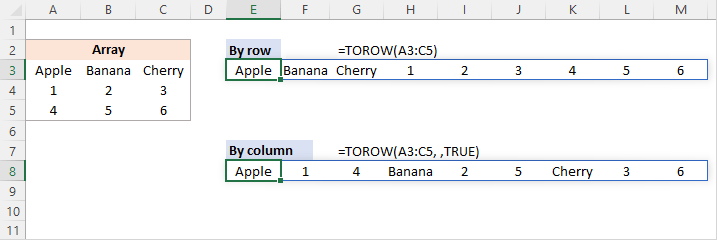## Merge multiple ranges into one row

To combine several non-adjacent ranges into a single row, you first stack them horizontally or vertically into a single array with the help of HSTACK or VSTACK, respectively, and then use the TOROW function to convert the combined array into a row.

### Stack arrays horizontally and convert by row

With the first range in A3:C4 and the second range in A8:C9, the below formula will stack the two ranges horizontally into a single array, and then transform it to a row reading the values from left to right. The result is in E3 in the image below.

`=TOROW(HSTACK(A3:C4, A8:C9))`

### Stack arrays horizontally and convert by column

To read the stacked array vertically from top to bottom, you set the 3rd argument of TOROW to TRUE as shown in E5 in the image below:

`=TOROW(HSTACK(A3:C4, A8:C9), ,TRUE)`

### Stack arrays vertically and convert by row

To append each subsequent array to the bottom of the previous array and read the combined array horizontally, the formula in E12 is:

`=TOROW(VSTACK(A3:C4, A8:C9))`

### Stack arrays vertically and convert by column

To add each subsequent array to the bottom of the previous one and scan the combined array vertically, the formula is:

`=TOROW(VSTACK(A3:C4, A8:C9), ,TRUE)`

To better understand the logic, observe the different order of values in the resulting arrays: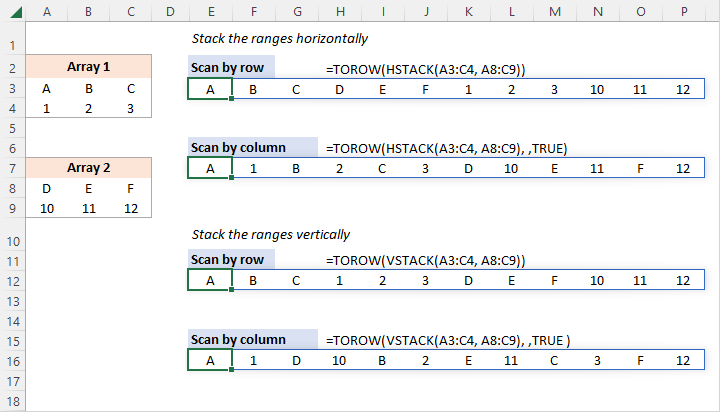## Extract unique values from a range into a row

Beginning with Microsoft Excel 2016, we have a wonderful function, named UNIQUE, that can easily get unique values from a single column or row. However, it cannot handle multi-column arrays. To overcome this limitation, use the UNIQUE and TOROW functions together.

For instance, to extract all the different (distinct) values from the range A2:C7 and place the results in one row, the formula is:

`=UNIQUE(TOROW(A2:C7), TRUE)`

As TOROW returns a one-dimensional horizontal array, we set the 2nd (by_col) argument of UNIQUE to TRUE to compare the columns against each other.

In case you want the results arranged in alphabetic order, wrap the above formula in the SORT function:

`=SORT(UNIQUE(TOROW(A2:C7), TRUE), , ,TRUE )`

As with UNIQUE, the by_col argument of SORT is also set to TRUE.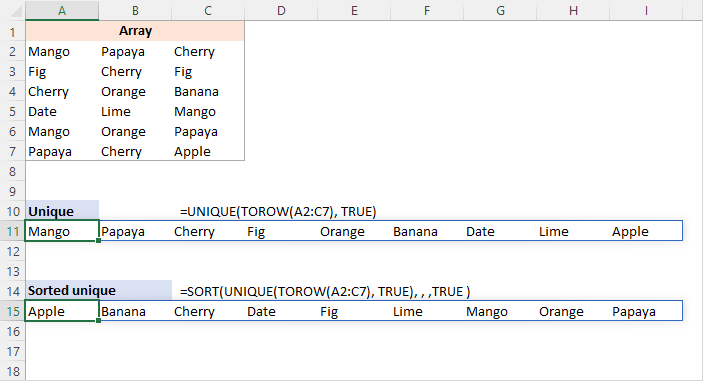## TOROW alternative for Excel 365 - 2010

In Excel versions where the TOROW function is not available, you can transform a range into a single row using a combination of a few different functions that work in older versions. These solutions are more complex, but they do work.

To scan the range horizontally, the generic formula is:

INDEX(range, QUOTIENT(COLUMN (A1)-1, COLUMNS(range))+1, MOD(COLUMN(A1)-1, COLUMNS(range))+1)

To scan the range vertically, the generic formula is:

INDEX(range, MOD(COLUMN(A1)-1, COLUMNS(range))+1, QUOTIENT(COLUMN (A1)-1, COLUMNS(range))+1)

For our sample dataset in A3:C5, the formulas take this shape:

To scan the range by row:

`=INDEX(\$A\$3:\$C\$5, QUOTIENT(COLUMN(A1)-1, COLUMNS(\$A\$3:\$C\$5))+1, MOD(COLUMN(A1)-1, COLUMNS(\$A\$3:\$C\$5))+1)`

This formula is an alternative to the TOROW function with the 3rd argument set to FALSE or omitted:

`=TOROW(A3:C5)`

To scan the range by column:

`=INDEX(\$A\$3:\$C\$5, MOD(COLUMN(A1)-1, COLUMNS(\$A\$3:\$C\$5))+1, QUOTIENT(COLUMN(A1)-1, COLUMNS(\$A\$3:\$C\$5))+1)`

This formula is equivalent to the TOROW function with the 3rd argument set to TRUE:

`=TOROW(A3:C5, ,TRUE)`

Please note that unlike the dynamic array TOROW function, these traditional formulas should be entered in each cell where you want the results to appear. In our case, the first formula (by row) goes to E3 and is copied through M3. The second formula (by column) lands in E8 and is dragged through M8.

For the formulas to copy correctly, we lock the range using absolute references (\$A\$3:\$C\$5). A named range will also do.

If you've copied the formulas to more cells than needed, a #REF! error will appear in "extra" cells. To fix this, wrap your formula in the IFERROR function like this:

`=IFERROR(INDEX(\$A\$3:\$C\$5, QUOTIENT(COLUMN(A1)-1, COLUMNS(\$A\$3:\$C\$5))+1, MOD(COLUMN(A1)-1, COLUMNS(\$A\$3:\$C\$5))+1), "")`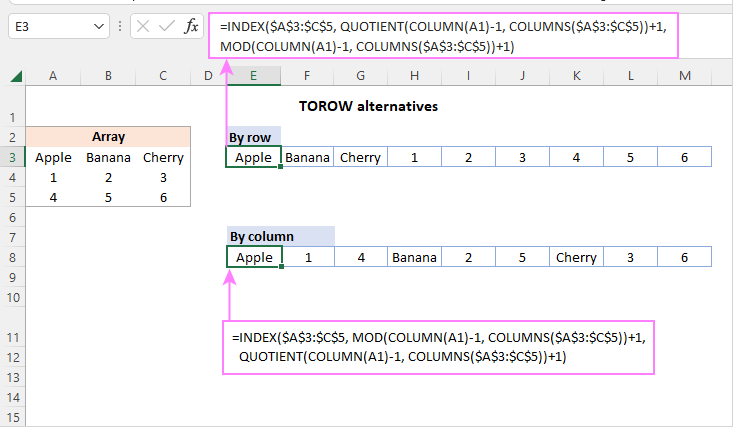How these formulas work

Below is a detailed break-down of the first formula that arranges the values by row:

`=INDEX(\$A\$3:\$C\$5, QUOTIENT(COLUMN(A1)-1, COLUMNS(\$A\$3:\$C\$5))+1, MOD(COLUMN(A1)-1, COLUMNS(\$A\$3:\$C\$5))+1)`

At the heart of the formula, we use the INDEX function to get the value of a cell based on its relative position in the range.

The row number is calculated by this formula:

`QUOTIENT(COLUMN(A1)-1, COLUMNS(\$A\$3:\$C\$5))+1`

The idea is to produce a repeating number sequence such as 1,1,1,2,2,2,3,3,3, … where each number repeats as many times as there are columns in the source range. And here's how we do this:

QUOTIENT returns the integer portion of a division.

For numerator, we use COLUMN(A1)-1, which returns a serial number from 0 in the first cell where the formula is entered to n (total number of values in the range minus 1) in the last cell where the formula in entered. In this example, we have 0 in E2 and 8 in M3.

For denominator, we use COLUMNS(\$A\$3:\$C\$5)). This returns a constant number equal to the number of columns in your range (3 in our case).

As a result, the QUOTIENT function returns 0 in the first 3 cells (E3:G3), to which we add 1, so the row number is 1.

For the next 3 cells (H3:J3), QUOTIENT returns 1, and +1 gives the row number 2. And so on.

To calculate the column number, you build an appropriate number sequence using the MOD function:

`MOD(COLUMN(A1)-1, COLUMNS(\$A\$3:\$C\$5))+1`

As there are 3 columns in our range, the sequence must look like: 1,2,3,1,2,3,…

The MOD function returns the remainder after division.

In E3, MOD(COLUMN(A1)-1, COLUMNS(\$A\$3:\$C\$5))+1

becomes

MOD(1-1, 3)+1)

and returns 1.

In F3, MOD(COLUMN(B1)-1, COLUMNS(\$A\$3:\$C\$5))+1

becomes

MOD(2-1, 3)+1)

and returns 2.

Once the row and column numbers are established, INDEX easily fetches the value at the intersection of that row and column.

In E3, INDEX(\$A\$3:\$C\$5, 1, 1) returns a value from the 1st row and the 1st column of the referenced range, i.e. from cell A3.

In F3, INDEX(\$A\$3:\$C\$5, 1, 2) returns a value from the 1st row and the 2nd column, i.e. from cell B3.

And so forth.

The second formula that scans the range by column, works in a similar way. The difference is that we use MOD to calculate the row number and QUOTIENT to figure out the column number.

## TOROW function not working

If the TOROW function results in an error, it's most likely to be one of these reasons:

### #NAME? error

With most Excel functions, a #NAME? error is a clear indication that the function's name is misspelled. With TOROW, it may also mean that the function is not available in your Excel. If your Excel version in other than 365, try using a TOROW alternative.

### #NUM error

A #NUM! error indicates that the returned array cannot fit into a row. Most often that occurs when you reference entire columns and/or rows instead of a smaller range.

### #SPILL error

In most cases, a #SPILL error suggests that the row where you've entered the formula does not have enough blank cells to spill the results into. If the neighboring cells are visually empty, make sure there are no spaces or other non-printing characters in them. For more information, see What #SPILL error means in Excel.

That's how you use the TOROW function in Excel to convert a 2-dimensional array or range into a single row. I thank you for reading and hope to see you on our blog next week!

Excel TOROW function - formula examples (.xlsx file)

## You may also be interested in

1. Hi Alexander,
how can I put in rows only the value on 2nd column grouped by value in first column
in example I have this table
Col_1 Col_2
fruit apple
fruit orange
fruit banana
meat veal
meat pork
And now I want it like this:
Col_1 Col_2 Col 3 Col_4
fruit Apple orange banana
meat veal Pork
I wouldn't care of the name of columns
but I have a huge file of 20K rows with in Col-1 around 5000 different values Col_2 all different but related only to one value in Col_1
what Tranposition can I use?